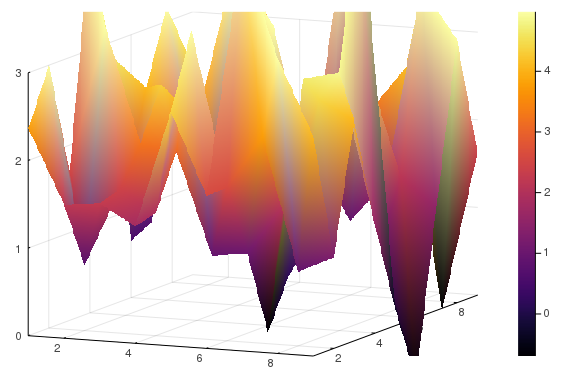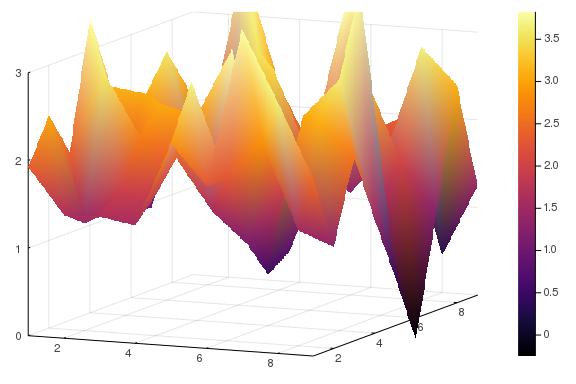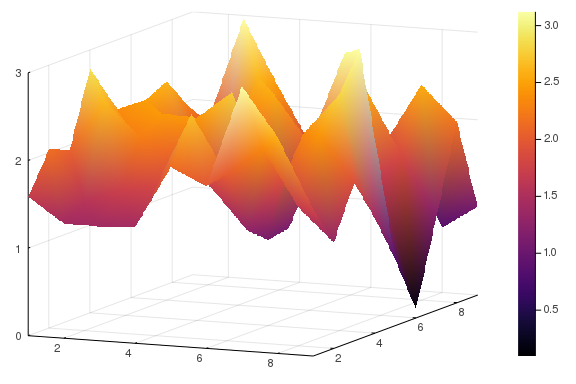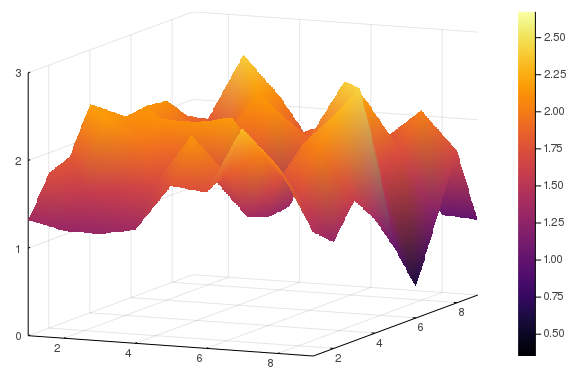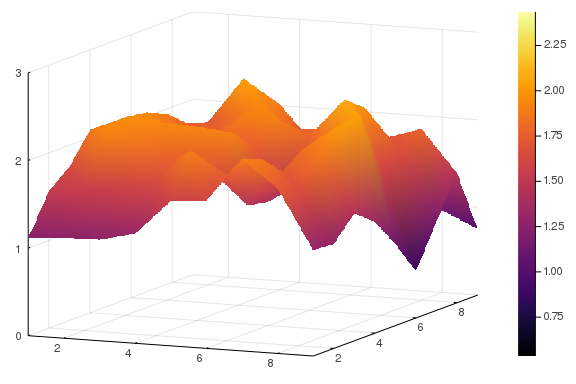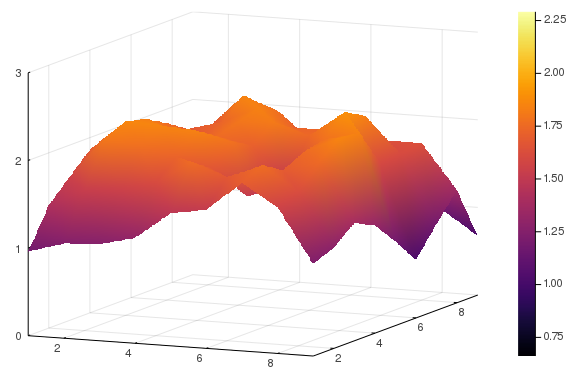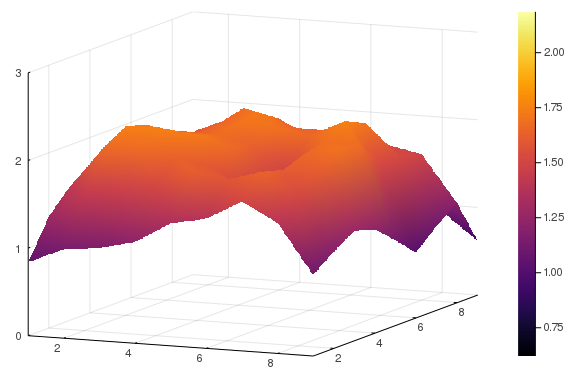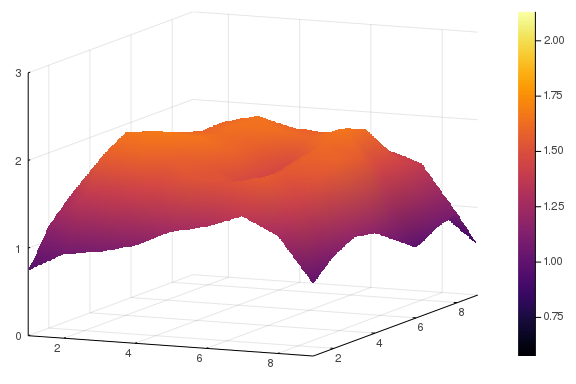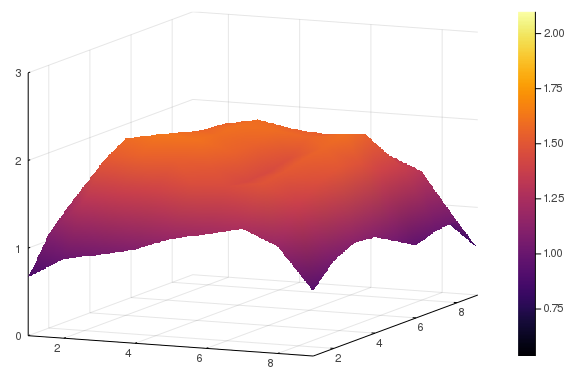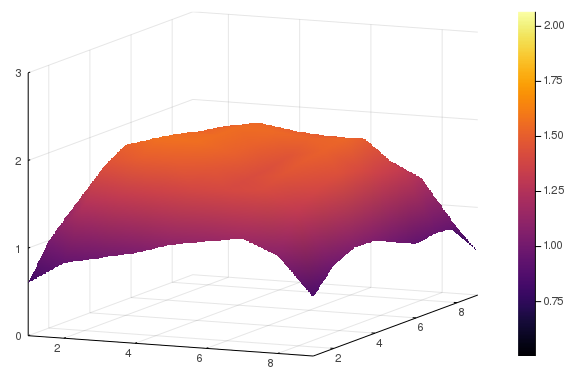# Numerically Solving Partial Differential Equations

##### January 6th, 2020

Let's continue our previous discussion. Last time we established a relationship between recurrent neural networks and their continuous form, the neural ordinary differential equation. After doing so, we noticed that convolutional neural networks are directly related to the derivative operators of a partial differential equation. Now, let's see how we would mathematically handle partial differential equations numerically, and understand what kind of issues we should expect when using them as our underlying modeling tool.

## Method of Lines Discretizations of Partial Differential Equations

### The one-dimensional heat equation

A method of lines discretization of a PDE is the transformation of that PDE into an ordinary differential equation. This is not a difficult process, in fact, it occurs simply when we leave one dimension of the PDE undiscretized. The canonical case for this is the heat equation, also known as the diffusion equation, given by the following PDE:

$u_t = \Delta u$

In one dimension, this is $u_t = u_{xx}$. Recall from last time that the second order discretization of the second derivative is the operation

$\frac{u(x+\Delta x)-2u(x)+u(x-\Delta x)}{\Delta x^{2}}=u^{\prime\prime}(x)+\mathcal{O}\left(\Delta x^{2}\right).$

Thus to discretize our PDE, let's take our domain, say, $x \in [0,1]$, and split it up by $\Delta x$ pieces. Thus instead of solving for the full continuous $u(x)$ at a given time, we just want to know $u_i = u(x_i)$ at each $x_i = i \Delta x$. This transforms the continuous problem to solving for $U(t)$ which is the array of $u_i$ at a given time point.

On this array, the second order central difference formula holds, which means that the second derivative operator can be written in terms of elements of our solution array:

$u_{xx} \approx \frac{u_{i-1} - 2 u_i + u_{i+1}}{\Delta x^2}$

and so we get the ODE system:

$u_i' = \frac{u_{i-1} - 2 u_i + u_{i+1}}{\Delta x^2}$

as our approximation to the PDE. We can also represent the operation as the tridiagonal matrix with [1 -2 1] down the diagonal, a matrix known as the Strang matrix, in which case we would have:

$U' = AU$

### Coding the one-dimensional heat equation

Let's give ourselves some random initial condition:

n = 9
u0 = randn(n) .+ 2.0

9-element Array{Float64,1}:
0.09312295741917254
0.3343919930253889
2.0345100924810593
1.0652676775022107
-0.21360617717287456
0.8722038798636851
2.2724758402880503
3.291617463646782
1.1229085263461545


Now we define the ODE system:

function heat_1d(du,u,p,t)
dx2 = (1/(length(u)+1))^2
for i in 2:(length(u)-1)
du[i] = (u[i-1] - 2u[i] + u[i+1])/dx2
end
du = (-2u + u)/dx2
du[end] = (u[end-1] - 2u[end])/dx2
end

heat_1d (generic function with 1 method)


Notice here that there was an extra little choice that was required: the boundary condition. With a convolutional stencil we always drop off the last values. In a partial differential equation, this is the choice to only solve on the interior and assume $u(0) = u(1) = 0$, which is known as the Dirichlet 0 boundary condition. More generally we can choose specific values at the end point to represent sources and sinks. Another popular choice of boundary condition are the Neumann boundary conditions, where $u'(0) = a$ is some chosen value. A popular choice is what's known as the no-flux boundary condition, which is where $u'(0)=0$ which implies that none of the fluid is able to flow over the boundary (the advection at the boundary is zero).

Here we are using Dirichlet zero boundary conditions, saying $u_0 = u_{n+1} = 0$. Now let's solve the ODE defined by this system and plot it over time:

using OrdinaryDiffEq
prob = ODEProblem(heat_1d, u0, (0.0,0.5))
sol = solve(prob,Tsit5(),saveat=0.05)

using Plots
plot(sol)
plot!(sol)
plot!(sol)
plot!(sol)
plot!(sol)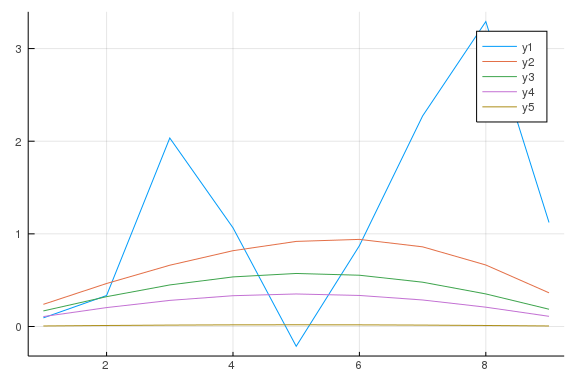Notice that the heat fluctuations quickly dampen out and then the heat is released from the system. This is because we do not have a source of heat and just have a way for it to leave our domain. Now let's say we have a source on the left hand side which is giving a value of 3, i.e. it's fixed at 30 degree celcius. We start with random heat in the room and let the thermal energy diffuse: how does the temperature at each part of the room change over time?

u0 = randn(n) .+ 2.0

function heat_1d_d3(du,u,p,t)
dx2 = (1/(length(u)+1))^2
for i in 2:(length(u)-1)
du[i] = (u[i-1] - 2u[i] + u[i+1])/dx2
end
du = (3.0 + -2u + u)/dx2
du[end] = (u[end-1] - 2u[end])/dx2
end

prob = ODEProblem(heat_1d_d3, u0, (0.0,0.5))
sol = solve(prob,Tsit5(),saveat=0.05)

plot(sol)
plot!(sol)
plot!(sol)
plot!(sol)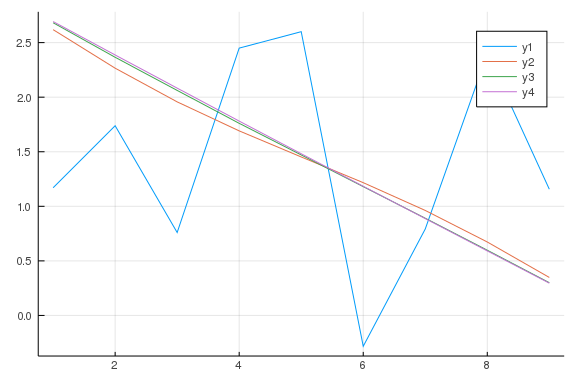Notice that we evolve towards a gradient from the source to the sink (you can prove that after infinite time you have a straight line from the source to the sink!).

### The two-dimensional heat equation

Now let's say we have $u_t = \Delta u$ where $\Delta u = u_{xx} + u_{yy}$. In this case, we do the same discretization along the $x$ and $y$ to get the stencil

0  1 0
1 -4 1
0  1 0

derived in the last class. To implement this, we can use a matrix representation over time that we evolve, like:

n = 9
u0 = randn(n,n) .+ 2.0

function heat_2d(du,u,p,t)
dx2 = (1/(size(u,1)+1))^2
for i in 2:(size(u,1)-1), j in 2:(size(u,2)-1)
du[i,j] = (u[i-1,j] + u[i,j-1] - 4u[i,j] + u[i+1,j] + u[i,j+1])/dx2
end
for i in 2:(size(u,1)-1)
du[1,i] = (u[1,i-1] -4u[1,i] + u[2,i] + u[1,i+1])/dx2
du[i,1] = (u[i-1,1] -4u[i,1] + u[i,2] + u[i+1,1])/dx2
du[end,i] = (u[end-1,i] - 4u[end,i] + u[end,i+1] + u[end,i-1])/dx2
du[i,end] = (u[i,end-1] - 4u[i,end] + u[i+1,end] + u[i-1,end])/dx2
end

du[1,1] = (u[2,1] + u[1,2] - 4u[1,1])/dx2
du[1,end] = (u[2,end] + u[1,end-1] - 4u[1,end])/dx2
du[end,1] = (u[end,2] + u[end-1,1] - 4u[end,1])/dx2
du[end,end] = (u[end-1,1] + u[end,end-1] - 4u[end,end])/dx2
end

prob = ODEProblem(heat_2d, u0, (0.0,0.01))
sol = solve(prob,Tsit5(),saveat=0.001)

surface(sol)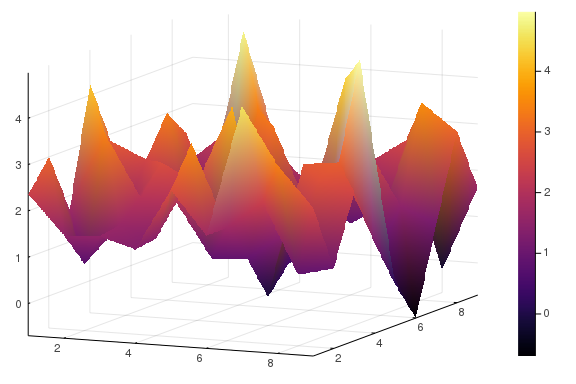surface(sol)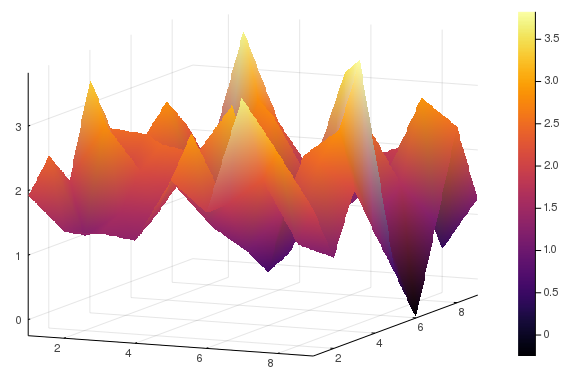surface(sol)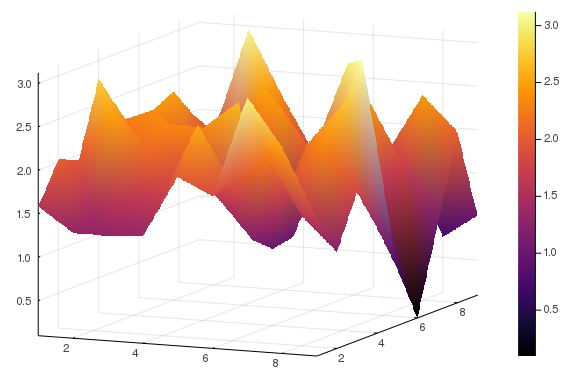for i in 1:length(sol)
display(surface(sol[i],zlims = (0.0,3.0)))
end